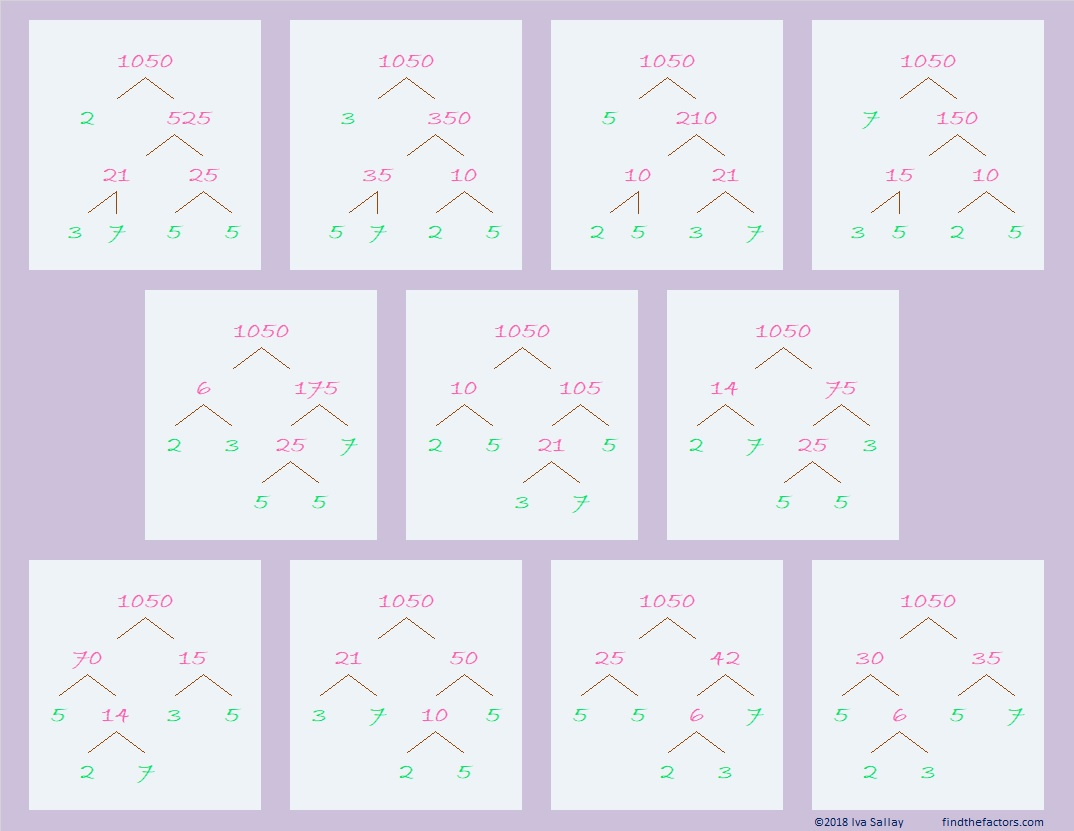# 1050 Factor Trees Are In Bloom This Spring

1050 has much more than the average number of factors for a number its size. I decided to make a few of its MANY possible factor trees in some springtime colors. I hope you enjoy looking at them. Its prime factors, 2, 3, 5, 5, and 7, are in green.What can I tell you about the number 1050?

• 1050 is a composite number.
• Prime factorization: 1050 = 2 × 3 × 5 × 5 × 7, which can be written 1050 = 2 × 3 × 5² × 7
• The exponents in the prime factorization are 1, 1, 2, and 1. Adding one to each and multiplying we get (1 + 1)(1 + 1)(2 + 1)(1 + 1) = 2 × 2 × 3 × 2 = 24. Therefore 1050 has exactly 24 factors.
• Factors of 1050: 1, 2, 3, 5, 6, 7, 10, 14, 15, 21, 25, 30, 35, 42, 50, 70, 75, 105, 150, 175, 210, 350, 525, 1050
• Factor pairs: 1050 = 1 × 1050, 2 × 525, 3 × 350, 5 × 210, 6 × 175, 7 × 150, 10 × 105, 14 × 75, 15 × 70, 21 × 50, 25 × 42, or 30 × 35
• Taking the factor pair with the largest square number factor, we get √1050 = (√25)(√42) = 5√42 ≈ 32.403703If you had \$10.50 in quarters, you would have 42 quarters because 42(25) = 1050
If you had \$10.50 in dimes, you would have 105 dimes because 105(10) = 1050
If you had \$10.50 in nickles, you would have 210 nickles because 210(5) = 1050

1050 is the sum of the twenty-two primes from 7 to 97.

1050 is the hypotenuse of two Pythagorean triples:
630-840-1050 which is (3-4-5) times 210
294-1008-1050 which is (7-24-25) times 42

1050 looks interesting to me when it is written in a couple of other bases:
It’s UU in BASE 34 (U is 30 base 10) because 30(34) + 30(1) = 30(35) = 1050,
and it’s U0 in BASE 35 because 30(35) = 1050